## Maximum Power Transfer Theorem

I am doing Prof.Nagendra Krishna Pura’s Basics electrical circuits course provided by NPTEL. In week 6, I learnt about maximum power transfer theorem.

states that – A resistive load, being connected to a DC network, receives maximum power when the load resistance is equal to the internal resistance known as (Thevenin’s equivalent resistance) of the source network as seen from the load terminals. The Maximum Power Transfer theorem is used to find the load resistance for which there would be the maximum amount of power transfer from the source to the load.

To verify this theorem, I have taken Vth as 10V and Rth as 5K ohms connected in series with the load resistance RL. Now I am varying the RL to get the maximum power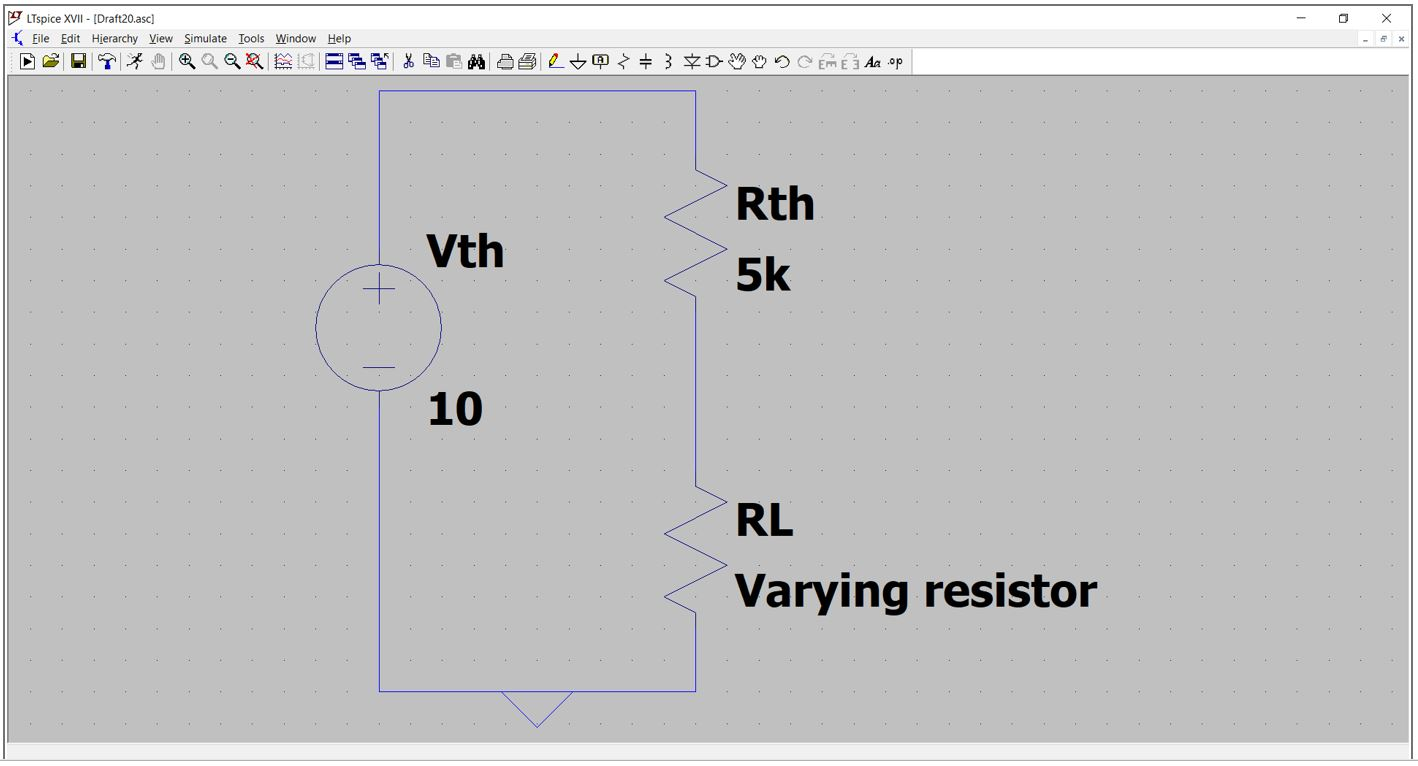For doing this I have written a python program and plotted the graph power(mW) vs RL(Kohms)

# Maximum power transfer thorem using pyplot

from matplotlib import pyplot as plt

Rth = 5e3

Vth = 10

RL = [x*1e3 for x in range(21) if x >0]

Vth_across_RL = [res/(Rth+res) for res in RL ]

I = [Vth*1e3/(Rth+res) for res in RL]

def power(Vtholtage_list,current_list):

power_list = []

for i in range(len(Vtholtage_list)):

p = Vtholtage_list[i]*current_list[i]

power_list.append(p)

return power_list

#print(power(Vth_across_RL, I))

power_list =[x*1e3  for x in power(Vth_across_RL, I)]

RL_kohms = [x/1e3 for x in RL]

plt.plot(RL_kohms,power_list)

plt.xlabel(“Resistance in Kohms”)

plt.ylabel(“Power in mW”)

plt.show()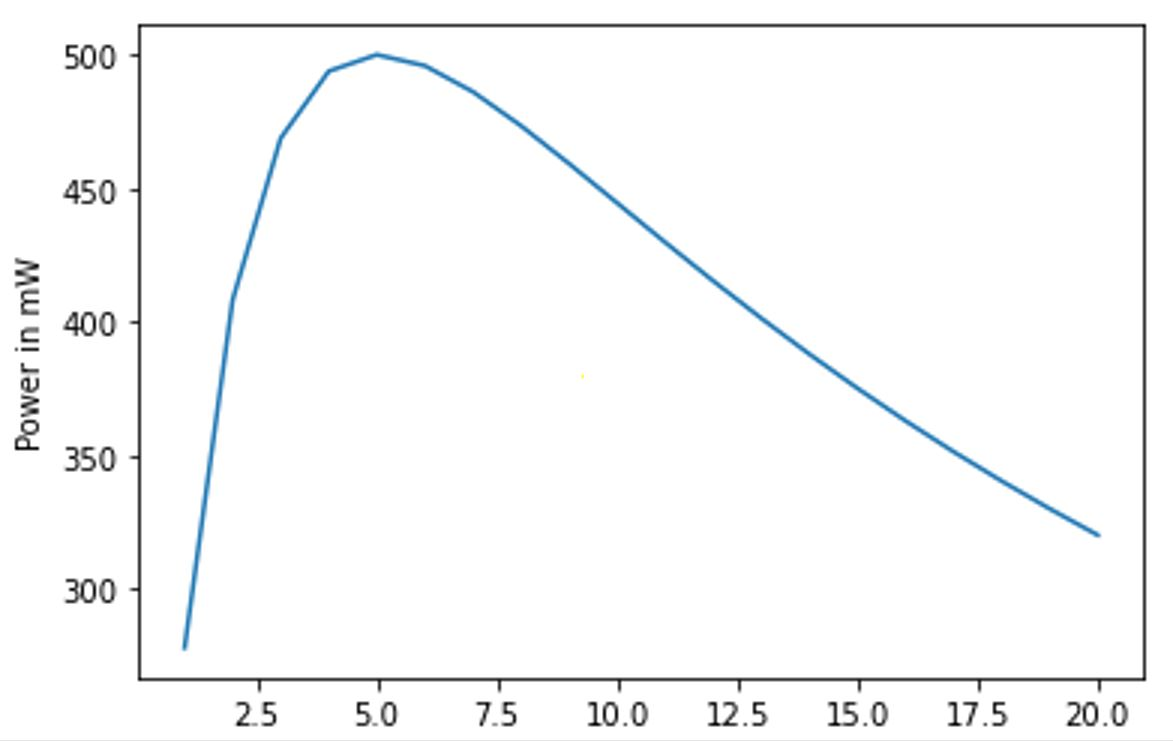From the graph I observed that when the load resistance (RL) is equal to the Thevenin resistance (Rth) of the circuits then I able to draw the maximum power from the source.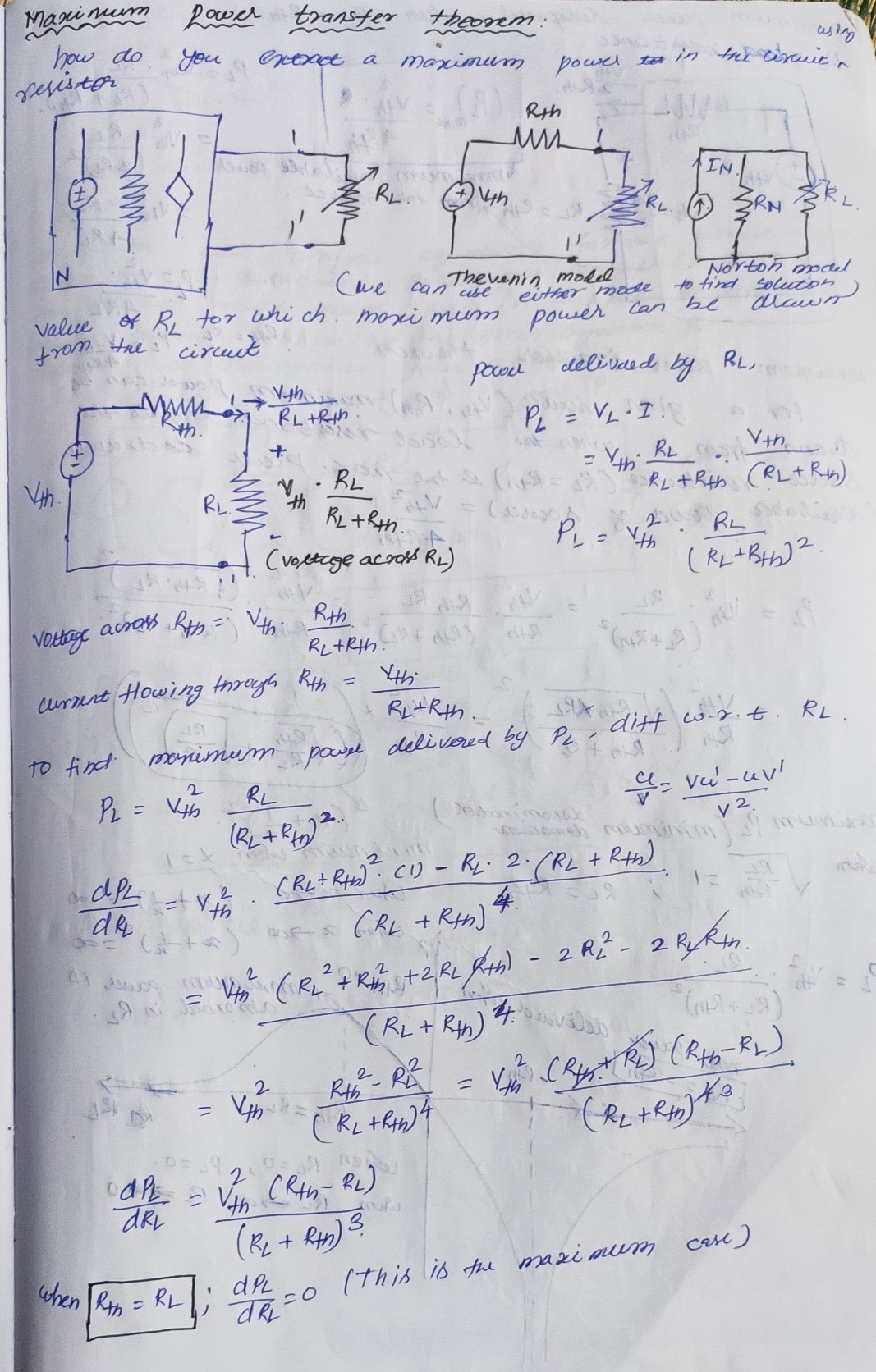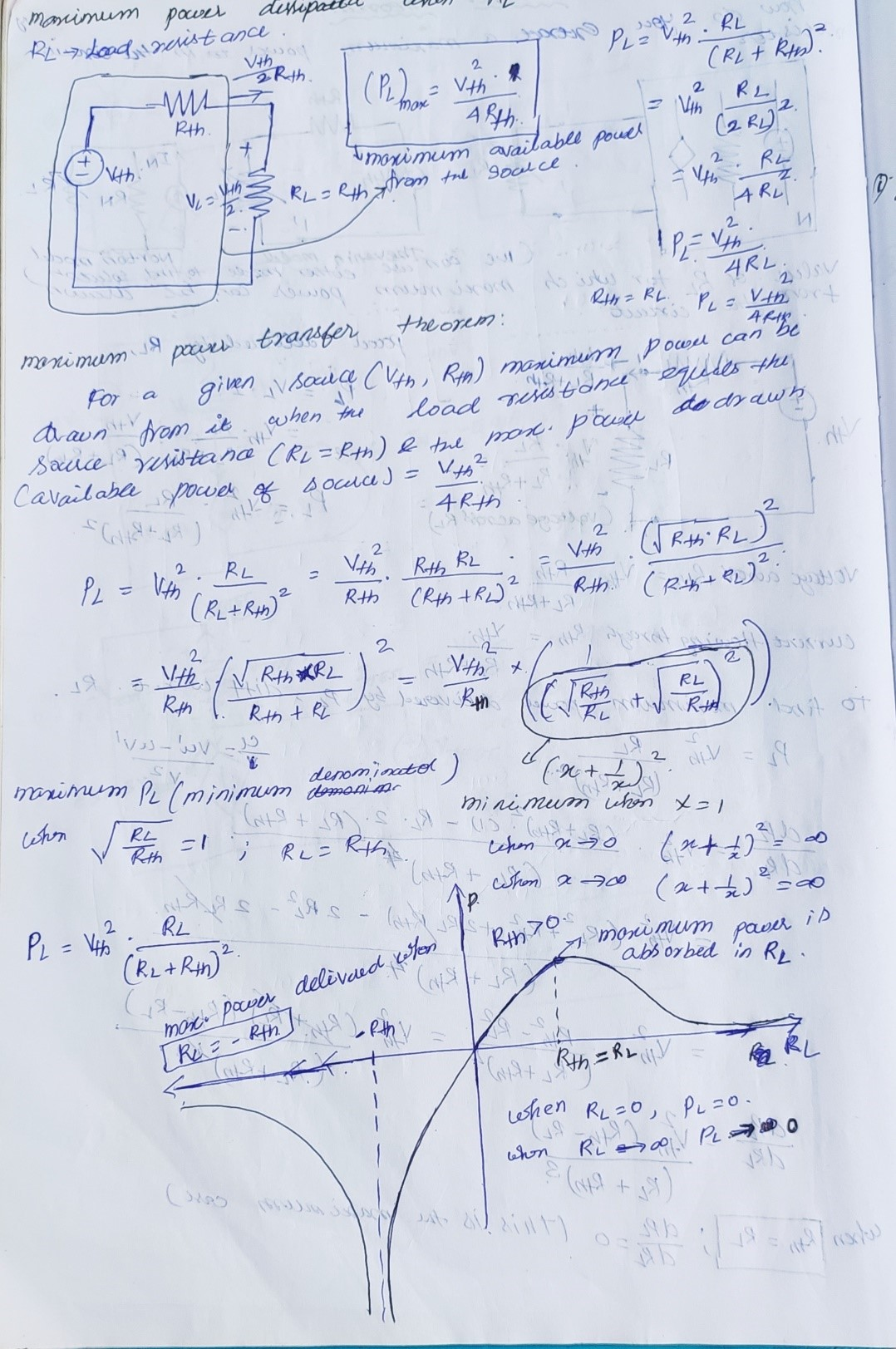## Interfacing BO Motor with Arduino

~ Vimal, Abilash, Prabha

We had learnt to interface a toy DC motor with the arduino platform. The idea is to design a sensor controlled vehicle which navigates based on the intensity of the light which falls on it.

We used a LDR(Light Dependent Resistor) as a sensor element for the project. The analog value of intensity of the light falling on it is measured and it ranges from 0 – 1024 (10 bit resolution limitation of arduino). We used a dual H bridge IC named L293D to actuate the motor. To get the desired torque, we have selected a geared motor with 300 RPM @ 12VDC.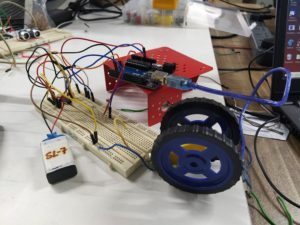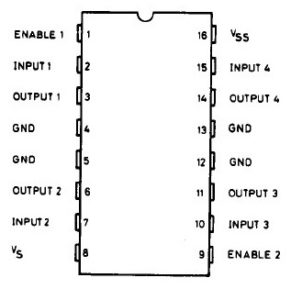For this task we used only one geared motor and one half of the dual H Bridge. For initial prototyping we used the breadboard, the enable pin of one half of the IC is connected to 5VDC, two IOs are assigned namely 4 and 7 to control the outputs of the IC. The LDR is connected to the analog pin A0. The LDR circuit is designed as a voltage divider circuit. The analog value obtained from the task is compared continuosly with the threshold limit of 950. If the value is changed the motor will rotate in clockwise direction else in anticlockwise direction.

## Automatic street light using LDR

~Ranjith, Abilash

While preparing for the electronics class. we took “Automatic street light using LDR” as the circuit, where “LED” turns ON in dark, and turns OFF in bright place.

After giving the circuit connection, the LED didn’t turn OFF when it was bright. So we started analyzing the circuit, then we found out that, the R1 resistor was low when compared to the resistance of the LDR under the brightness.

We calculated the resistor value of R1, by assuming that

1. Vbe = 0.6v was needed to turn ON the “transistor 1”.
2. Resistance of LDR = 180 kΩ under minimum light for which LED should be OFF (if we further reduced the light, resistance will increase, then “LED” should turn ON and “transistor 1” should turns OFF).

From the calculation, we got that R1 = 13.17 kΩ, we approximate it to 10 kΩ.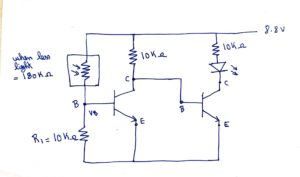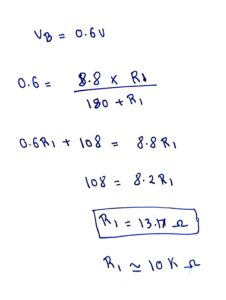Finally we made it to work.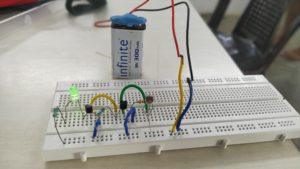## Turning ON/OFF light bulb using Arduino and Relay

~ Abilash, Pratap.

Abilash and Saranya made a relay circuit during the Electronic session with Vimal, so the goal was to turn ON and OFF the AC bulb with the delay of 1 second which was connected to the Arduino and Relay. The Arduino was connected to the laptop and bulb to the 230v Alternating Current through the relay.  Pratap captured the video as a sudden surprise and made the learning rigorous.

## Finch: using distance sensor

-Arun, Abilash

Abilash and I were testing the distance sensors in Finch Robot, which lead us to do a simple project where the Finch has to go through a track we made.

## Raspberry pi course

In STEM land a person from Auroville came to the iSMART class to conduct a course about raspberry pi course. Initially I dont know how to use raspberry pi. I was curious to learn raspberry pi. I also learnt the differences and advantages between Arduino and Raspberry pi. I learnt the different pin functions and the number of pins on a raspberry pi board. Day 1&2 was fully theory session which was boring.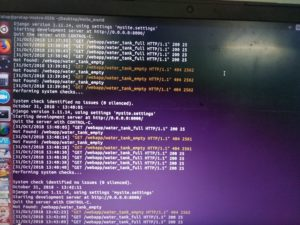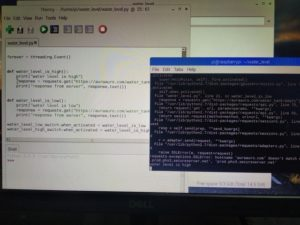In order to make it interesting I started ask many question to the teacher and the session was engaged by the participants. What really was interesting in the course was that the Arduino can’t drive a relay but with a raspberry pie we can drive a relay. One the teacher told that a relay can be driven by rapberry, he had a relay module but he couldn’t drive it. I took as a challenge and struggled for two days and I figured it to drive a relay. We made a small circuit with 2 LED where when the relay is on one of that LED will turn on and when the relay is off the other LED will turn on.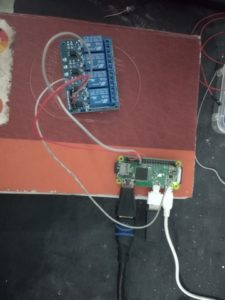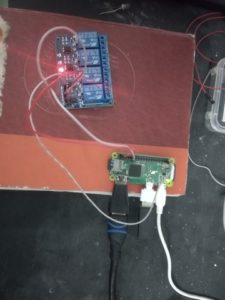After this the teacher was also very happy and he also learnt something from us. Then the next challenge was that to send and to receive inputs from a raspberry pi to a web page.The teacher had asked who knows web page development.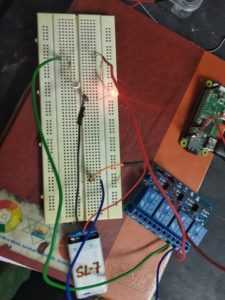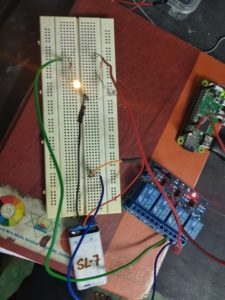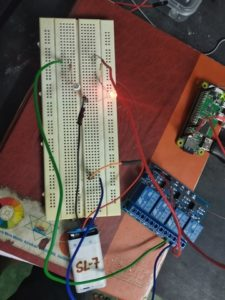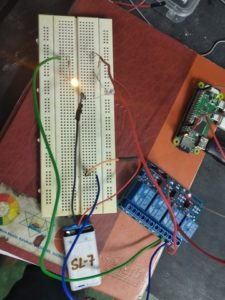Since I learnt Django web framework in STEM land, I was able to connect What I knew with what I lean now.  So I had asked him to show an example how to pass inputs from raspberry to a web page. Then finally in the last class we were able to complete all the task that we planed to accomplish in the course. Now I have some knowledge about raspberry pi and also I can build something with it.

## How to find a value of Capacitor

A capacitor is a two terminal passive element which stores energy.

There are two types of capacitor.

1. Ceramic Capacitor:

– These are small,non-polarised in yellow or orangish  in color.

How to identify these capacitor values:

The value is printed in three-digit code. The first two digits are the two most significant digits of the value, and the third digit is the exponent on the 10. The value is expressed in terms of pico-Farads.

For example,

104 becomes “10” followed by “0000”(four zeros) or 100000 pF, which can be written as 100 nF.2. Electrolytic Capacitor

– larger cylindrical bodies that look like small soda cans

– higher capacitance than ceramic capacitor

– they are polarized

How to identify these capacitor values and its polarization:

The value is followed by the voltage rating(maximum DC voltage the capacitor can withstand without damage).

Polarization:

There are two polarity indicators on an electrolytic cap:

1. The stripe painted on the body usually denotes the negative lead.

For example,

1000uf 25V – Thousand micr farads and 25 volt.

## designing 4-bit microprocessor using magic tool

I started designing 4-bit microprocessor in magic tool, it consists of three block:

• register to store an input data.
• 4-xor gates and one carrier input is used to 2’s complement for subtraction

Inputs which are used in it :

• two 4-bit data A and B
• carrier input
• clock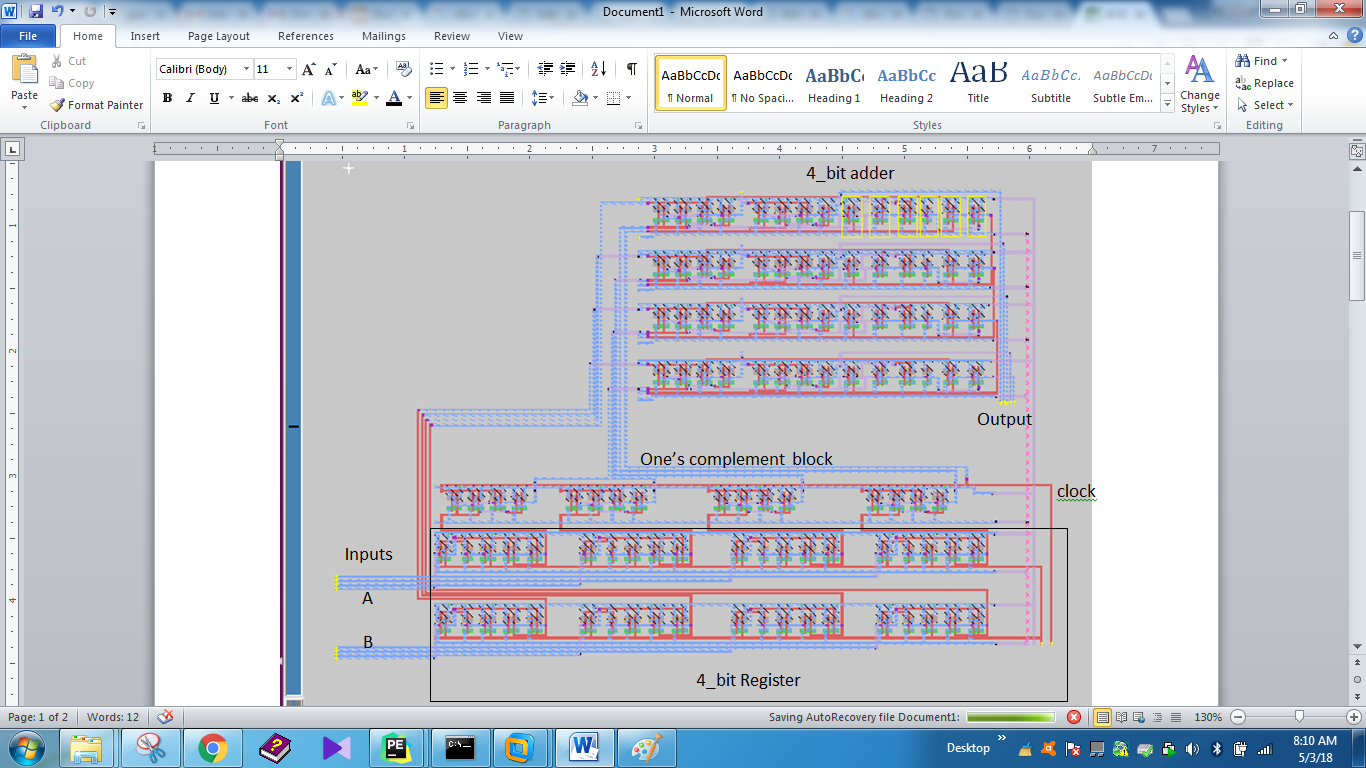In this microprocessor, the adding operation is takes place when carrier input is 0, and the subtraction operation takes place when carrier input is 1. During subtraction, one’s complement block takes one’s complement B data and it send to 4_bit adder. The carrier input value is 1 at subtraction. so it’s form two’s complement of B data in 4-bit full adder.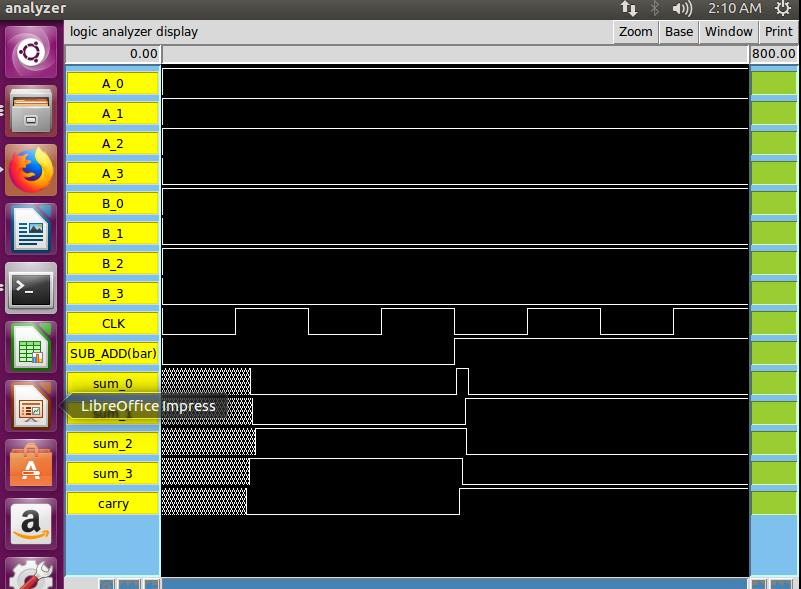And also sanjeev taugth me, to align block in order to reduce area and to create data bus with multiplexing two inputs.

## First digit on clock

– Logeshwari

In electronics, initially I displayed my name using Seven Segment Display(SSD). I had already posted that in My First Blog. After that I wanted to learn more on SSD. So I was asked to make a digital clock. I have completed the first digit of the seconds. I used two JK flip flops (HD74LS76A), decoder (SN74LS47), a SSD (Common Anode) and Signal Generator. I first made the connections of JK flip flop on a breadboard. The input is clock signal which is generated from a signal generator of Frequency 1Hz. The combination of the two flip flops acts like a counter. Once the circuit is connected, I tested the output of the flip flop using an ocilloscope. I got the waveforms like this.

Then I took the decoder and connected the outputs to the input pins of the decoder. Decoder (converts the binary information to 2n outputs) is connected to the seven segment display. While doing decoder I was able to recall Karnaugh-Map which is the logic behind it. The output of the decoder is fed to the seven segment display. Once everything is done then it counts from zero to fifteen (f-represented in binary).

Since the one’s digit of the clock counts only upto nine and after that it should reset to zero. For resettting the digit to zero I used NAND gate. Binary representation of nine is 1001. When the Most Significant Bit and the Least Significant Bit are high(1) then it should reset to zero.

I have completed the first step only, but I feel happy that I learnt and understood what I did. I acknowledge Sanjeev, Sundhar and Ranjith for guiding me.

## Sinthanai Sangamam with around 600 teachers(Azim Premji Foundation)

Saranya

Sinthanai Sangamam is happened on 21/02/2018. It is a new kind of experience with around 600 teachers from different places. I have presented a paper about STEM land. The great thing is I got an opportunity to share about, my journey with the children in STEM land.The wonderful thing is that I am in STEM land. Why I came to STEM land is, I want to learn more about electronics that is why I came to STEM. I have learned about electronics what I have learnt that came as a few product that is inverter, display my name using 7-segment display, mobile charger, solar powered charger etc..Then I learned some of the programming from students that is scratch and geo gebra these program will be used in mathematics. These things I have explained in very shortly. In Sinthanai Sangamam everyone shared their experience with the children. How they interact with the children, what method they are following in their school, what kind of projects their students done and how we can connect to science and maths in our real life. The one main thing what I learnt is there should not a gap between children and teacher.

In VLSI layout course I learned to design a gates using NAND. Then I have checked the NAND gate truth table with the help of bread board, battery and multimeter. I learned to use magic tool, how to extract and how to simulate the layout.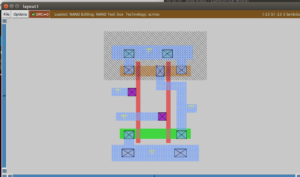I learned the cross section of CMOS and what colour coding we have to use for the each components.